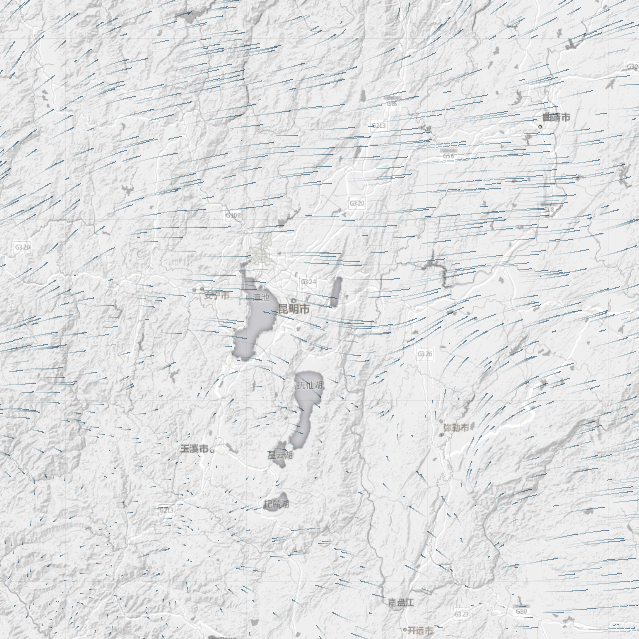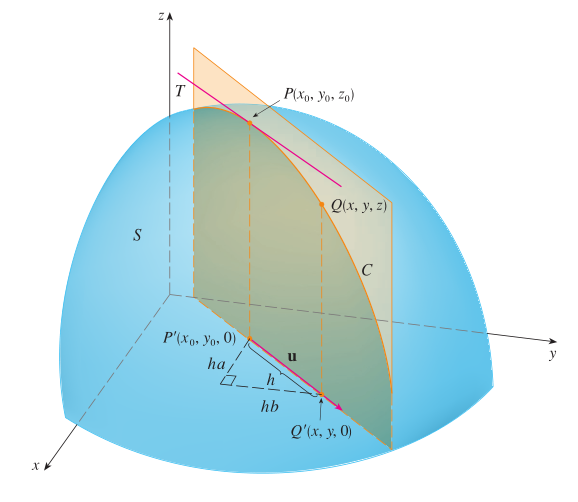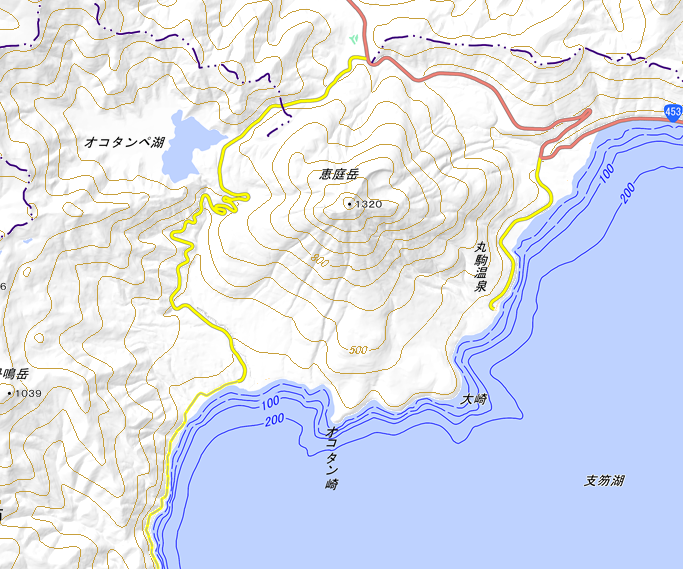Site Overlay

# 矢量微积分：1. 向量场、梯度场

## 向量场$\bold{F} = P\bold{i}+Q\bold{j}$$$\mathbf{E}(x, y, z)=kQ\left(\frac{x}{\left(x^{2}+y^{2}+z^{2}\right)^{3 / 2}} \mathbf{i}+\frac{y}{\left(x^{2}+y^{2}+z^{2}\right)^{3 / 2}} \mathbf{j}+\frac{z}{\left(x^{2}+y^{2}+z^{2}\right)^{3 / 2}} \mathbf{k}\right)$$

## 梯度场

### 梯度

$$\begin{array}{l} f_{x}\left(x_{0}, y_{0}\right)=\lim _{h \rightarrow 0} \frac{f\left(x_{0}+h, y_{0}\right)-f\left(x_{0}, y_{0}\right)}{h} \\ f_{y}\left(x_{0}, y_{0}\right)=\lim _{h \rightarrow 0} \frac{f\left(x_{0}, y_{0}+h\right)-f\left(x_{0}, y_{0}\right)}{h} \end{array}$$

（下面这段话就是想求面上一点的切线斜率）$h\to 0$，就得到了 z$$\bold{u}方向上的的变化率，记作 D_{\bold{u}}f(x_0,y_0)，称为f(x_0,y_0)方向导数。定义式：$$ D_{\mathrm{u}} f\left(x_{0}, y_{0}\right)=\lim _{h \rightarrow 0} \frac{f\left(x_{0}+h a, y_{0}+h b\right)-f\left(x_{0}, y_{0}\right)}{h} $$偏导数正是方向导数的特殊情况——方向正好是轴向。 如果有方向导数，对于这个方向的方向向量\mathbf{u}=\langle a, b\rangle，有：$$ D_{\mathrm{u}} f(x, y)=f_{x}(x, y) a+f_{y}(x, y) b $$那么这个函数 f(x,y) 可微。由于 \bold{u} 是单位向量，所以可以用三角换元得到：$$ D_{\mathfrak{u}} f(x, y)=f_{x}(x, y) \cos \theta+f_{y}(x, y) \sin \theta $$### 梯度向量 梯度就是梯度向量 梯度相当于一种多元函数的导数 根据点积的定义，可以把方向导数表示为$$ \begin{aligned} D_{\mathfrak{u}} f(x, y) &=f_{x}(x, y) a+f_{y}(x, y) b \\ &=\left\langle f_{x}(x, y), f_{y}(x, y)\right\rangle \cdot\langle a, b\rangle \\ &=\left\langle f_{x}(x, y), f_{y}(x, y)\right\rangle \cdot \mathbf{u} \end{aligned} $$\left\langle f_{x}(x, y), f_{y}(x, y)\right\rangle 就是梯度向量，记作 \nabla f。它其实就是这点的法向量。 梯度向量的计算公式：$$ \nabla f(x, y)=\left\langle f_{x}(x, y), f_{y}(x, y)\right\rangle=\frac{\partial f}{\partial x} \mathbf{i}+\frac{\partial f}{\partial y} \mathbf{j} $$梯度向量与此处单位方向导数的积就是此处的方向导数。$$ D_{\mathfrak{u}} f(x, y)=\nabla f(x, y) \cdot \mathbf{u} $$学习线性代数的时候， 我们常常看见这样的平面方程：$$ \frac{x+2}{\color{blue}-1}=\frac{y-1}{\color{blue}2}=\frac{z+3}{\color{blue}-\frac{2}{3}} $$向量 \langle-1,2,-\dfrac{2}{3}\rangle 正是梯度向量。 【例子】计算 f(x, y)=x^{2} y^{3}-4 y$$(2,-1) 的方向导数。已知方向向量为：$\mathbf{v}=2 \mathbf{i}+5 \mathbf{j}$

【解】首先计算 $(2,-1)$ 处的梯度向量：

\begin{aligned}\nabla f(x, y) &=2 x y^{3} \mathbf{i}+\left(3 x^{2} y^{2}-4\right) \mathbf{j} \\\nabla f(2,-1) &=-4 \mathbf{i}+8 \mathbf{j}\end{aligned}

$$\mathbf{u}=\frac{\mathbf{v}}{|\mathbf{v}|}=\frac{2}{\sqrt{29}} \mathbf{i}+\frac{5}{\sqrt{29}} \mathbf{j}$$

\begin{aligned}D_{\mathfrak{u}} f(2,-1) &=\nabla f(2,-1) \cdot \mathbf{u}=(-4 \mathbf{i}+8 \mathbf{j}) \cdot\left(\frac{2}{\sqrt{29}} \mathbf{i}+\frac{5}{\sqrt{29}} \mathbf{j}\right) \\&=\frac{-4 \cdot 2+8 \cdot 5}{\sqrt{29}}=\frac{32}{\sqrt{29}}\end{aligned}

### 什么是数量场？### 势场### 什么是梯度场？

$$\nabla f(x, y) =2 x y^{3} \mathbf{i}+\left(3 x^{2} y^{2}-4\right) \mathbf{j}$$